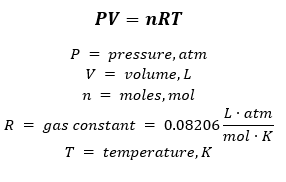# Problem: Dry nitrogen gas is bubbled through liquid benzene (C 6H6) at 20.0 ˚C. From 100.0 L of the gaseous mixture of nitrogen and benzene, 24.7 g benzene is condensed by passing the mixture through a trap at a temperature where nitrogen is gaseous and the vapor pressure of benzene is negligible. What is the vapor pressure of benzene at 20.0 ˚C?

###### FREE Expert Solution

To calculate the vapor pressure of benzene (C6H6), we’re going to use the ideal gas equation:Given:

Mass C6H6 = 24.7 g
Temperature = 20.0°C +273.15 K = 293.15 K
V = 100.0 L

Calculate moles C6H6:

91% (224 ratings)###### Problem Details

Dry nitrogen gas is bubbled through liquid benzene (C 6H6) at 20.0 ˚C. From 100.0 L of the gaseous mixture of nitrogen and benzene, 24.7 g benzene is condensed by passing the mixture through a trap at a temperature where nitrogen is gaseous and the vapor pressure of benzene is negligible. What is the vapor pressure of benzene at 20.0 ˚C?